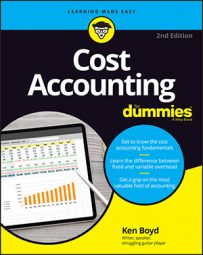##### Cost Accounting For DummiesWhen cost accounting, you can allocate costs at different levels: unit level, batch level, and so forth. A batch is a group of units, just like a batch of cookies you bake in the oven. You can analyze how you produce batches (for example, batches of a simple, high-volume product compared to batches of a complex, low-volume product) and possibly reduce your costs. That’s better than spreading costs uniformly, which can result in distortions.

Say you make glass bottles. You’re in a great environment for cost allocation by batch. A machine shapes the glass into bottles and imprints your logo. Your static budget assumes that the cost of loading and unloading bottles is allocated based on a batch of 50 bottles. Here is an analysis of your loading costs.

 Actual Static Bottles produced (A) 10,000 12,000 Batch size (B) 50 48 Batches produced (A × B) 200 250 Labor hours per batch 3 2.5 Total labor hours (C) 600 625 Cost per labor hour (D) \$22 \$21 Total labor costs (C × D) \$13,200 \$13,125

You have just traced labor costs at a different level in cost hierarchy. Instead of tracing costs to a unit, you’ve traced costs to a batch.

It’s not hard to apply flexible budget techniques to a batch. When you know how to apply the per-unit static budget amount to actual production, it’s a short step to applying the per-batch static budget amount to actual production.

This table calculates flexible budget cost.

 Actual units 10,000 Divide by static batch size 48 Equals number of batches 208 Multiply by static labor hours per batch 2.5 Equals flexible budget labor hours 521 Multiply by static labor rate per hour \$21 Equals flexible budget labor costs \$10,938

The best way to reduce costs is to analyze multiple variances (in fact, analyze as much data as you can). You see labor price and efficiency variances in tables earlier in the chapter. Now apply the concepts at the batch level. These tables show a labor price variance calculation and a labor efficiency variance.

Glass Bottles — Labor Price Variance
Actual Quantity × Actual Price Actual Quantity × Budgeted Price
600 hours 600 hours
Multiply by \$22/hour Multiply by \$21/hour
Equals \$13,200 (A) Equals \$12,600 (B)
Labor price variance (A – B) \$600

Because the price variance relates to cost, the negative variance of \$625 is favorable. You spent less than planned in the static budget.

Glass Bottles — Labor Efficiency Variance
Actual Quantity × Budgeted Price Budgeted Quantity × Budgeted Price
600 hours 625 hours
Multiply by \$21/hour Multiply by \$21/hour
Equals \$12,600 (A) Equals \$13,125 (B)
Labor efficiency variance (A – B) -\$525 Favorable

The negative labor cost variance is favorable. You spent less than planned. Now calculate the flexible budget variance:

```Flexible budget variance = price variance + efficiency variance
Flexible budget variance = \$600 + -\$525
Flexible budget variance = \$75```